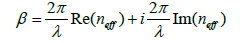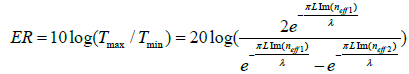## Research Journal of Optics and Photonics

All submissions of the EM system will be redirected to Online Manuscript Submission System. Authors are requested to submit articles directly to Online Manuscript Submission System of respective journal.

Research Article, Res J Opt Photonics Vol: 2 Issue: 1

# The Graphene MachZehnder Modulator Based on Sandwiched Structure

Feng Zhou1* and Wei Du2

1Department of Information Science and Electronic Engineering, Zhejiang University of Media and Communications, Hangzhou, China, 310018

2Department of Computer Science and Computer Engineering, University of Arkansas, Fayetteville, United States of America 70701

*Corresponding Author : Feng Zhou
Department of Information Science and Electronic Engineering, Zhejiang University of Media and Communications, Hangzhou, 310018, China
E-mail: 20150029@zjicm.edu.cn

Received: February 8, 2018 Accepted: February 09, 2018 Published: February 16, 2018

Citation: Zhou F, Du W (2018) The Graphene Mach-Zehnder Modulator Based on Sandwiched Structure. Res J Opt Photonics 2:1.

## Abstract

A Graphene Mach-Zehnder modulator based on sandwiched structure is proposed where bi-layer graphene is inserted inside the silicon waveguide to enhance the modulation ability. The calculated results show that the modulator exhibits an excellent performance in terms of small footprint and high extinction ratio. Besides, the modulation ability of the waveguide remains stable under the variations of the temperature and the wavelength of the incident light.

## Introduction

Optical modulators featuring compact size, high modulation speed, large modulation depth and low energy consumption are much appreciated in the application of optical devices. Based on the modulation mechanisms, modulators can be classified into phase, absorption and polarized modulators  which are usually made of silicon materials. However, since the electro-optic effects are too weak in silicon, conventional modulators are usually limited by a narrow operation bandwidth, a relatively low transmission speed and a large footprint [2,3], which makes it impossible to be integrated on chip. To overcome those limitations, graphene-based modulators have been intensively investigated due to its unprecedented properties such as enhanced interaction with light, high carrier mobility and gate-controllable conductivity [4-6]. For example, Liu has reported a 25 μm long electro-absorption modulator by placing a monolayer graphene on the top of silicon waveguide . However, electroabsorption modulators are still limited by high energy consumption and low modulation depth.

In this article, we have demonstrated an ultra-compact graphene Mach-Zehnder modulator by inserting bi-layer graphene into the center of silicon waveguide to form the sandwiched structure. The expected slot effect and bi-layer graphene design has made significant enhancement on the modulation ability of whole silicon waveguide.

## Operation Principle

For an isolated graphene sheet, the chemical potential is determined by the carrier density, which can be controlled by applying a gate voltage onto the graphene. The according chemical potential will lead to a variation of the graphene’s permittivity which can be illustrated by the Kubo formula , Figure 1(a) and 1(b) show the conductivity and the permittivity as a function of chemical potential, respectively. The blue and red curves represent the real and imaginary parts for these parameters. It should be noted in Figure 1(b) that since graphene is treated as a kind of anisotropic materials, the permittivity is only in the two-dimensional lattice plane and its in-plane permittivity can be actively tuned by the chemical potential while its out-of-plane permittivity is a constant of 2.5 that is same as graphite .

Figure 1: The optical properties of graphene as a function of chemical potential: (a) conductivity of graphene under different chemical potentials; (b) permittivity of graphene under different chemical potentials. Results are obtained from the Kubo formula of an infinite graphene sheet at the wavelength of 1550 nm.

According to the semi-classical model, the interlayer coupling can be ignored and the conductivity of bi-layer can be assumed to be δbi = 2δmono . The thickness of the bi-layer graphene can be estimated at d1 = 2Δd, thus the permittivity of bi-layer graphene is basically the same as monolayer graphene. Since the surface contact area of bi-layer graphene and light are larger than that of monolayer graphene and light, as well as the formed sandwiched structure can enhance the light-matter interaction as discussed in reference , the optical interaction between light and graphene is expected to be further enhanced by utilizing the sandwiched structure and bi-layer graphene.

To demonstrate the above assumption, a bi-layer graphene sandwiched waveguide structure is constructed here shown as Figure 2 (a). From the previous analysis it is known that by adjusting the applied bias voltages on the bi-layer graphene, the chemical potential can be tuned which in turn can cause the change of the effective mode index of the whole structure. The electric field distribution of the waveguide for the TE mode is shown as Figure 2 (b). It can be seen that most of the light tends to be concentrated on the center of the waveguide.

Figure 2: (a) The schematic view of the waveguide; (b) the electric field distribution of the waveguide for TE mode at μc =0 eV; (c) the simulation and calculation results of Re(neff); (d) the simulation and calculation results of Im(neff).

The effective mode index neff of the structure for TE modes under the change of chemical potential from 0 eV to 1 eV are shown in Figure 2 (c), 2(d), including the real part Re (neff) and imaginary part Im(neff). It is noticed that Re (neff) reaches the largest value 1.935 at μc = 0.4 eV, and the minimum value of Re (neff) is 1.912 at μc = 1 eV. Thus the effective mode index variation Δneff of the whole waveguide is calculated to be 0.023. For the bi-layer graphene embedded waveguide, the phase of the incident light will have a change through a certain length in the waveguide which can be expressed by the formula Δφ = (2π /λ)× Δneff × L. A required length for phase change π is only 33.5 mm for the proposed structure. Compared with conventional silicon waveguide, the proposed structure can greatly enhance the phase modulation ability over 100 times and make the size reduced to a dozens of micros.

Based on the above simulation results, a Mach-Zehnder modulator with small footprint based on the proposed sandwiched structure is configured here. The schematic picture of Mach-Zehnder modulator based on 6 bi-layer graphene sheets embedded silicon waveguide is shown as Figure 3(a) and 3(b).

Figure 3: The vertical view of the proposed Mach-Zehnder modulator; (b) the schematic view of the Mach-Zehnder modulator.

The graphene sheet can be grown by the chemical vapor deposition (CVD) method and transferred on the silicon-on-insulator (SOI) substrate, and electrodes can be positioned to connect with bilayer graphene and silicon layer. According to interference theory, the transmission of the proposed Mach-Zehnder modulator can be expressed as :(1)

where Δφ represents the phase difference of the two arms, β1, β2 are the propagation constants of the two arms, respectively. The propagation constant β is deduced from the effective mode index which can be obtained by the simulation software:(2)

Equation 1 shows that when Δφ = 0, which implies the phases of two arms are the same, the output energy of the Mach-Zehnder modulator reaches the maximum value Tmax, and this state is called ON state (signal “1”). Similarly, when Δφ = 0, when phases of two arms are exactly reversed, the output energy of the Mach-Zehnder modulator reaches the minimum value Tmin which is called OFF state (signal “0”). The extinction ratio (ER), also known as the modulation depth, is an important parameter to measure the performance of the proposed Mach-Zehnder modulator, which can be defined as :(3)

where neff1 and neff2 are the effective mode index for ON and OFF state, respectively.

At μc1 = 1 eV, the attenuation (Im(neff )) has the smallest value, which stands for ON state, therefore the chemical potential can be fixed at 1 eV for the first arm (unmodulated arm). For OFF state, a different chemical potential μc2 must be set for the second arm (modulated arm), and the phase difference between the two arms will reach π after propagating through a required length. For example, the required length to achieve π shift is 14.3 μm if we set μc2 = 0.6 eV for the second arm. As we all know, neff has an according variation under the variation of the chemical potential, thus the required length to realize π phase shift under different chemicals of the modulated arm is also changed shown as the black line in Figure 4, and the minimum length of the arm is 7 μm when μc2 = 0.39 eV. Figure 4 also depicts the ER variation of the proposed Mach-Zehnder modulator under different chemical potentials, it can be seen that ER reaches the peak of 31 dB at μc2 = 0.58 eV. Moreover, at μc2 = 0.58 eV, the transmission of the modulator for ON state is 92.2% while the transmission is only 0.06% for OFF state. Furthermore, if the width of the arm is 400 nm, the distance between electrodes is 2200 nm and the width of the electrode is 700 nm, the footprint of the modulator can be achieved with the minimal value of 35 μm2.

Figure 4: Red line depicts the extinction ratio as a function of the chemical potential μc2 and black line depicts the required length to realize π phase shift as a function of chemical potential μc2.

## Conclusion

We have proposed a Mach-Zehnder modulator with greatly enhanced modulation ability by inserting bi-layer graphene inside the silicon waveguide. The major advantages of the proposed modulator include the small footprint (35 μm2) and high extinction ratio (31 dB). All of these advantages may promote applications for optical interconnects and optical integrated circuits in the future.

## References